# Normal Forms for CFGs Eliminating Useless Variables Removing

• Slides: 36Normal Forms for CFG’s Eliminating Useless Variables Removing Epsilon Removing Unit Productions Chomsky Normal Form 1Variables That Derive Nothing u. Consider: S -> AB, A -> a. A | a, B -> AB u. Although A derives all strings of a’s, B derives no terminal strings (can you prove this fact? ). u. Thus, S derives nothing, and the language is empty. 2Testing Whether a Variable Derives Some Terminal String u. Basis: If there is a production A -> w, where w has no variables, then A derives a terminal string. u. Induction: If there is a production A -> , where consists only of terminals and variables known to derive a terminal string, then A derives a terminal string. 3Testing – (2) u. Eventually, we can find no more variables. u. An easy induction on the order in which variables are discovered shows that each one truly derives a terminal string. u. Conversely, any variable that derives a terminal string will be discovered by this algorithm. 4Proof of Converse u. The proof is an induction on the height of the least-height parse tree by which a variable A derives a terminal string. A u. Basis: Height = 1. Tree looks like: u. Then the basis of the algorithm a 1. . . tells us that A will be discovered. an 5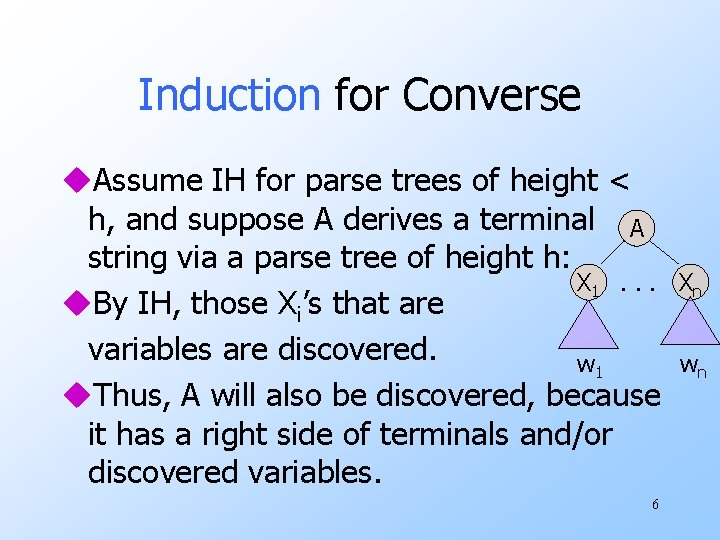Induction for Converse u. Assume IH for parse trees of height < h, and suppose A derives a terminal A string via a parse tree of height h: X 1. . . u. By IH, those Xi’s that are variables are discovered. w 1 u. Thus, A will also be discovered, because it has a right side of terminals and/or discovered variables. 6 Xn wn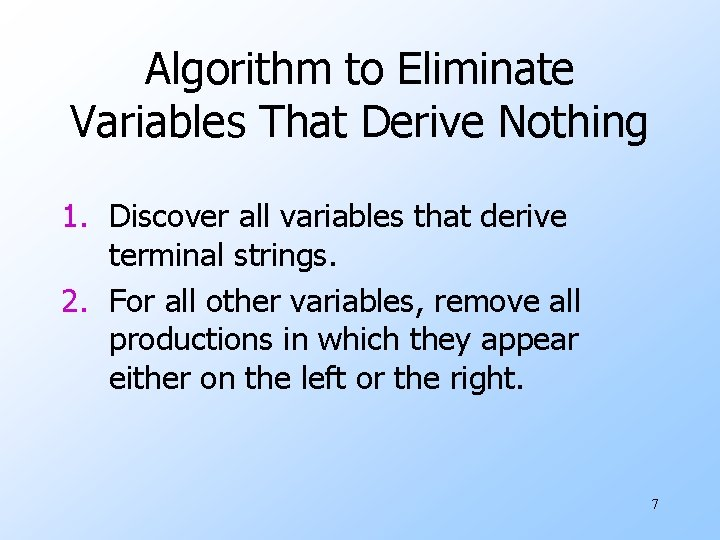Algorithm to Eliminate Variables That Derive Nothing 1. Discover all variables that derive terminal strings. 2. For all other variables, remove all productions in which they appear either on the left or the right. 7Example: Eliminate Variables S -> AB | C, A -> a. A | a, B -> b. B, C -> c u Basis: A and C are identified because of A -> a and C -> c. u Induction: S is identified because of S -> C. u Nothing else can be identified. u Result: S -> C, A -> a. A | a, C -> c 8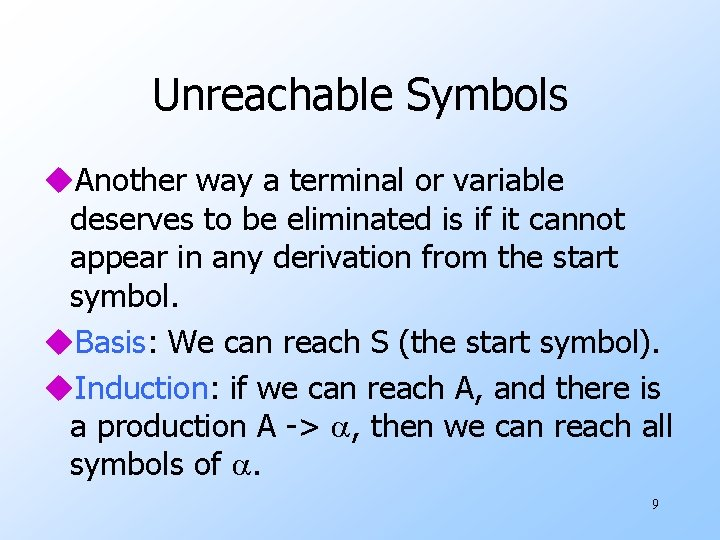Unreachable Symbols u. Another way a terminal or variable deserves to be eliminated is if it cannot appear in any derivation from the start symbol. u. Basis: We can reach S (the start symbol). u. Induction: if we can reach A, and there is a production A -> , then we can reach all symbols of . 9Unreachable Symbols – (2) u. Easy inductions in both directions show that when we can discover no more symbols, then we have all and only the symbols that appear in derivations from S. u. Algorithm: Remove from the grammar all symbols not discovered reachable from S and all productions that involve these symbols. 10Eliminating Useless Symbols u A symbol is useful if it appears in some derivation of some terminal string from the start symbol. u Otherwise, it is useless. Eliminate all useless symbols by: 1. Eliminate symbols that derive no terminal string. 2. Eliminate unreachable symbols. 11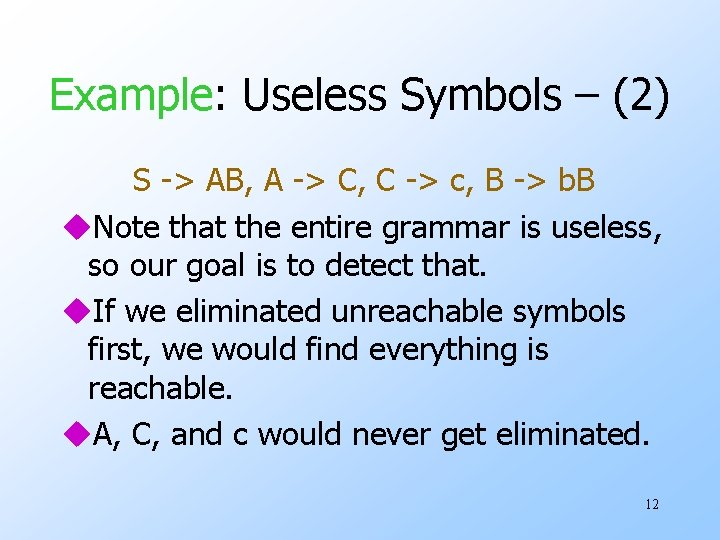Example: Useless Symbols – (2) S -> AB, A -> C, C -> c, B -> b. B u. Note that the entire grammar is useless, so our goal is to detect that. u. If we eliminated unreachable symbols first, we would find everything is reachable. u. A, C, and c would never get eliminated. 12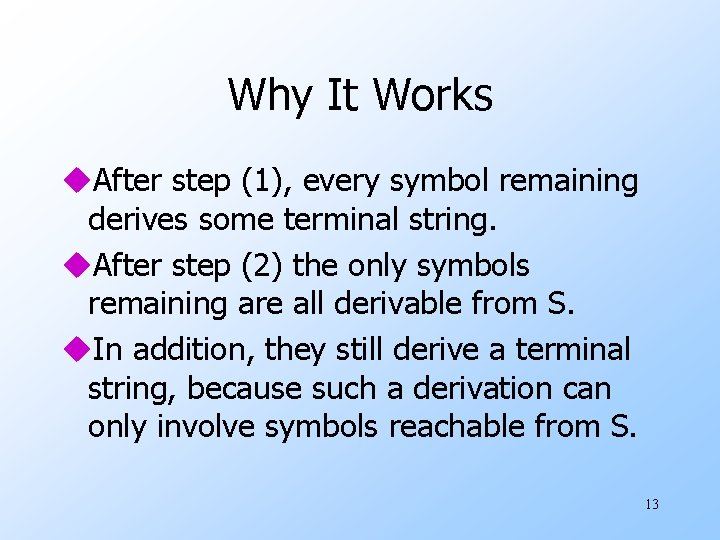Why It Works u. After step (1), every symbol remaining derives some terminal string. u. After step (2) the only symbols remaining are all derivable from S. u. In addition, they still derive a terminal string, because such a derivation can only involve symbols reachable from S. 13Epsilon Productions u. We can almost avoid using productions of the form A -> ε (called ε-productions ). w The problem is that ε cannot be in the language of any grammar that has no ε– productions. u. Theorem: If L is a CFL, then L-{ε} has a CFG with no ε-productions. 14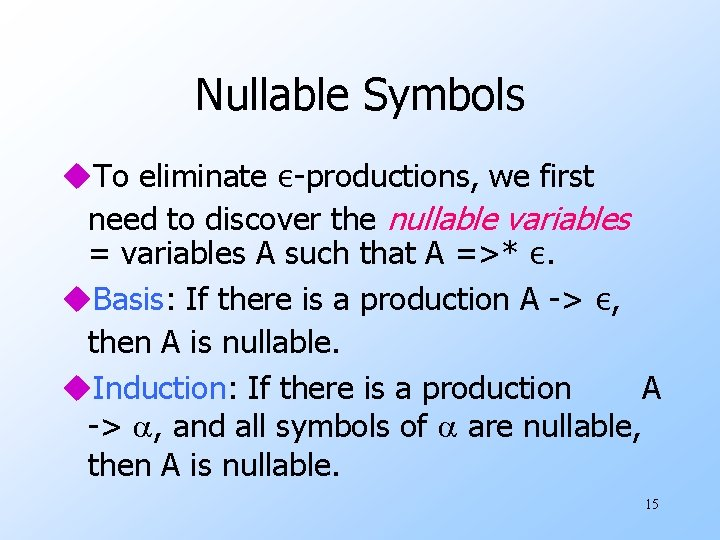Nullable Symbols u. To eliminate ε-productions, we first need to discover the nullable variables = variables A such that A =>* ε. u. Basis: If there is a production A -> ε, then A is nullable. u. Induction: If there is a production A -> , and all symbols of are nullable, then A is nullable. 15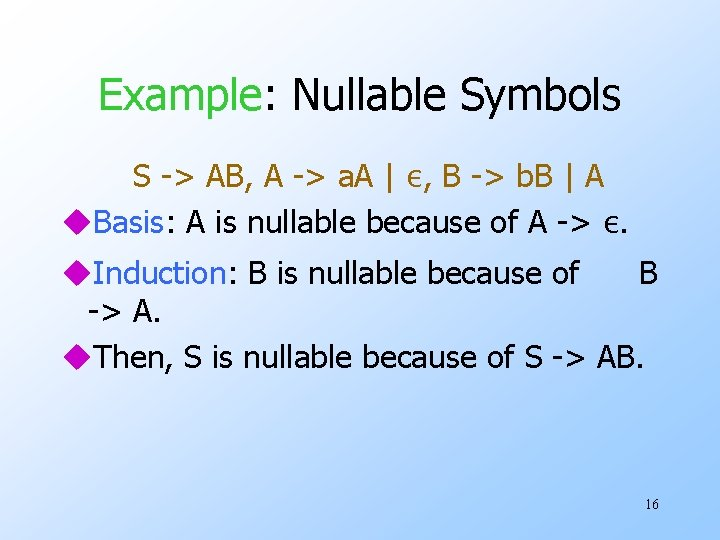Example: Nullable Symbols S -> AB, A -> a. A | ε, B -> b. B | A u. Basis: A is nullable because of A -> ε. u. Induction: B is nullable because of B -> A. u. Then, S is nullable because of S -> AB. 16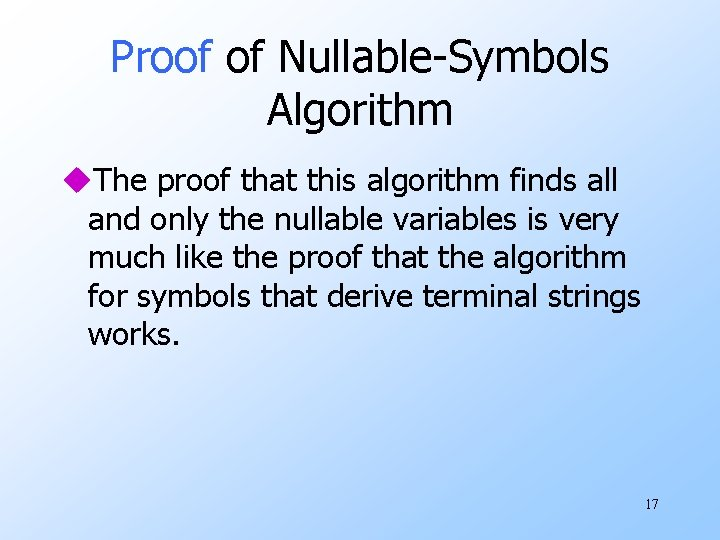Proof of Nullable-Symbols Algorithm u. The proof that this algorithm finds all and only the nullable variables is very much like the proof that the algorithm for symbols that derive terminal strings works. 17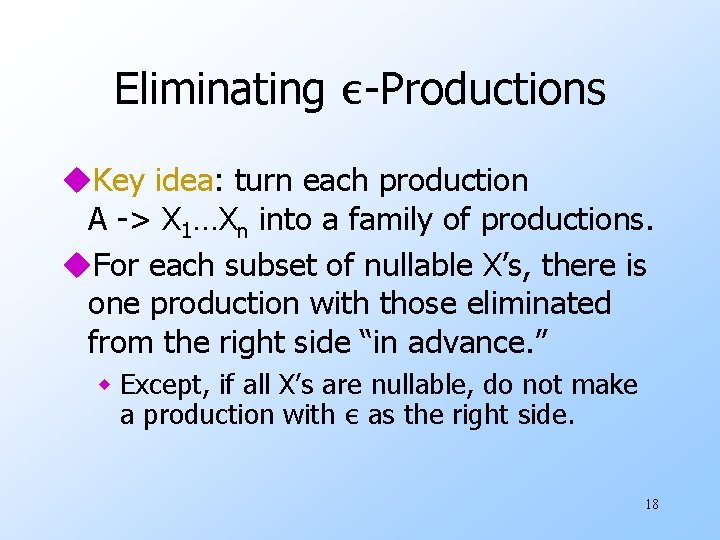Eliminating ε-Productions u. Key idea: turn each production A -> X 1…Xn into a family of productions. u. For each subset of nullable X’s, there is one production with those eliminated from the right side “in advance. ” w Except, if all X’s are nullable, do not make a production with ε as the right side. 18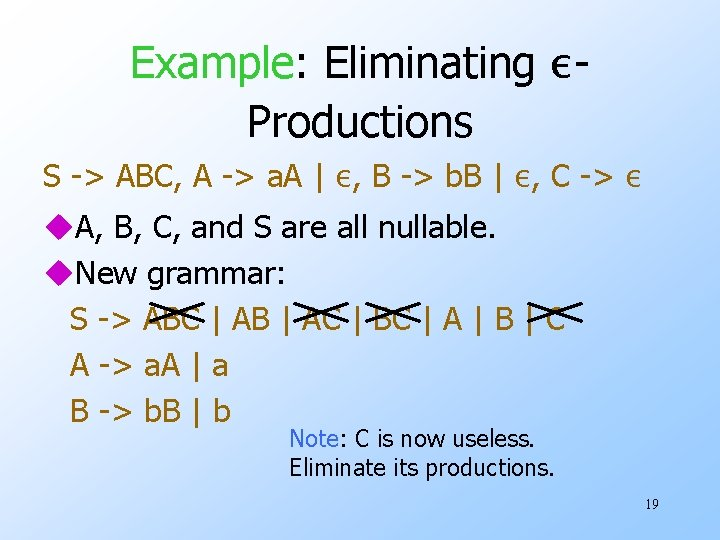Example: Eliminating εProductions S -> ABC, A -> a. A | ε, B -> b. B | ε, C -> ε u. A, B, C, and S are all nullable. u. New grammar: S -> ABC | AB | AC | BC | A | B | C A -> a. A | a B -> b. B | b Note: C is now useless. Eliminate its productions. 19Why it Works u Prove that for all variables A: 1. If w ε and A =>*old w, then A =>*new w. 2. If A =>*new w then w ε and A =>*old w. u Then, letting A be the start symbol proves that L(new) = L(old) – {ε}. u (1) is an induction on the number of steps by which A derives w in the old grammar. 20Proof of 1 – Basis u. If the old derivation is one step, then A -> w must be a production. u. Since w ε, this production also appears in the new grammar. u. Thus, A =>new w. 21Proof of 1 – Induction u. Let A =>*old w be an n-step derivation, and assume the IH for derivations of less than n steps. u. Let the first step be A =>old X 1…Xn. u. Then w can be broken into w = w 1…wn, uwhere Xi =>*old wi, for all i, in fewer than n steps. 22Induction – Continued u. By the IH, if wi ε, then Xi =>*new wi. u. Also, the new grammar has a production with A on the left, and just those Xi’s on the right such that wi ε. w Note: they all can’t be ε, because w ε. u. Follow a use of this production by the derivations Xi =>*new wi to show that A derives w in the new grammar. 23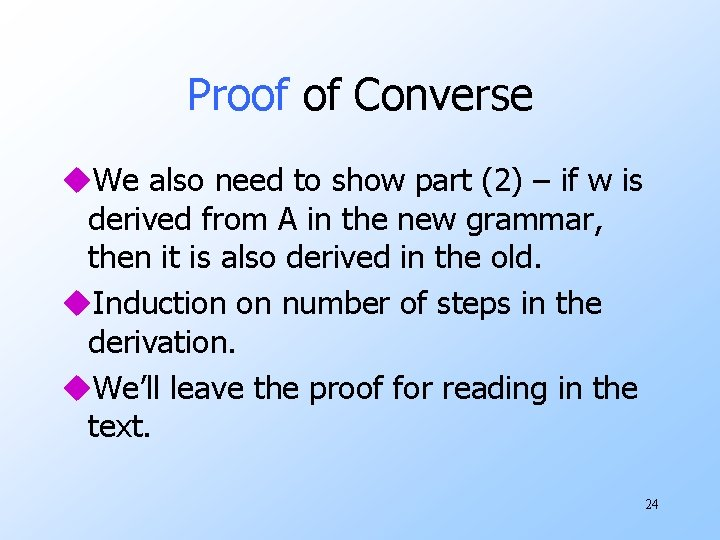Proof of Converse u. We also need to show part (2) – if w is derived from A in the new grammar, then it is also derived in the old. u. Induction on number of steps in the derivation. u. We’ll leave the proof for reading in the text. 24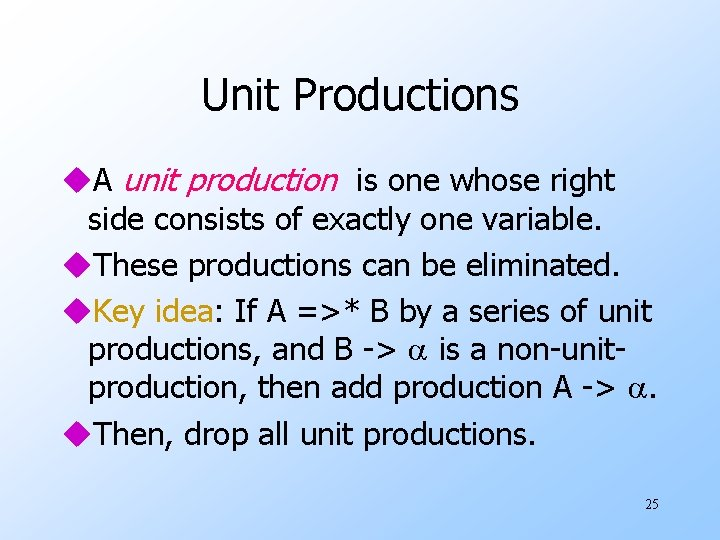Unit Productions u. A unit production is one whose right side consists of exactly one variable. u. These productions can be eliminated. u. Key idea: If A =>* B by a series of unit productions, and B -> is a non-unitproduction, then add production A -> . u. Then, drop all unit productions. 25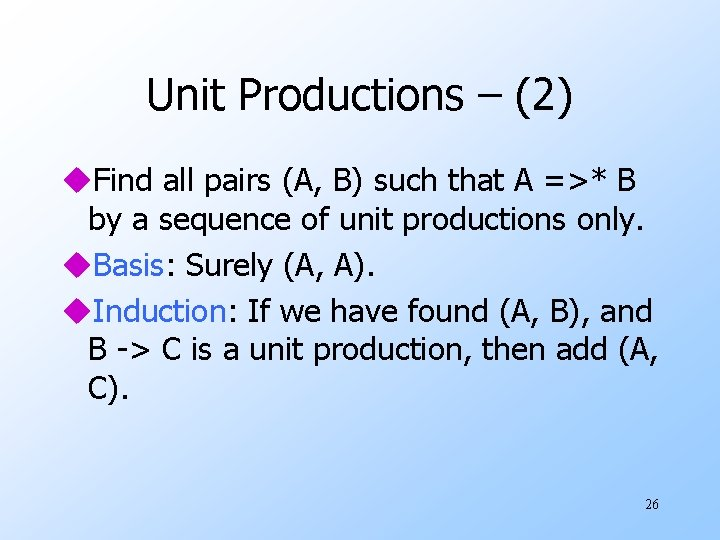Unit Productions – (2) u. Find all pairs (A, B) such that A =>* B by a sequence of unit productions only. u. Basis: Surely (A, A). u. Induction: If we have found (A, B), and B -> C is a unit production, then add (A, C). 26Proof That We Find Exactly the Right Pairs u. By induction on the order in which pairs (A, B) are found, we can show A =>* B by unit productions. u. Conversely, by induction on the number of steps in the derivation by unit productions of A =>* B, we can show that the pair (A, B) is discovered. 27Proof The the Unit-Production. Elimination Algorithm Works u. Basic idea: there is a leftmost derivation A =>*lm w in the new grammar if and only if there is such a derivation in the old. u. A sequence of unit productions and a non-unit production is collapsed into a single production of the new grammar. 28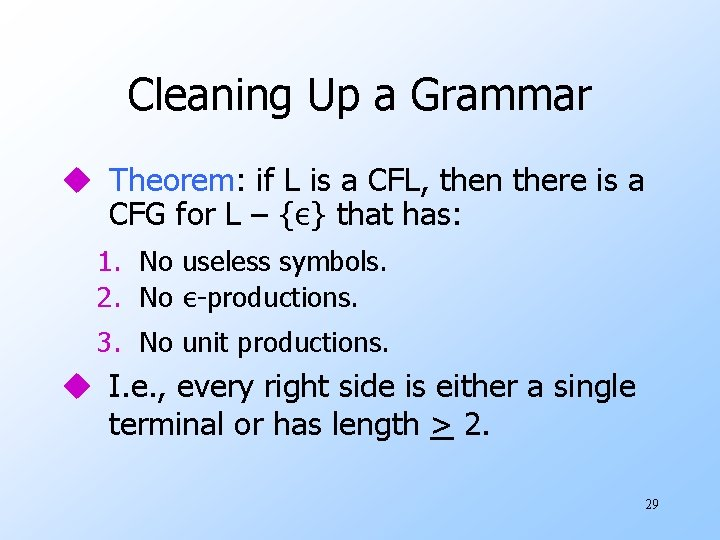Cleaning Up a Grammar u Theorem: if L is a CFL, then there is a CFG for L – {ε} that has: 1. No useless symbols. 2. No ε-productions. 3. No unit productions. u I. e. , every right side is either a single terminal or has length > 2. 29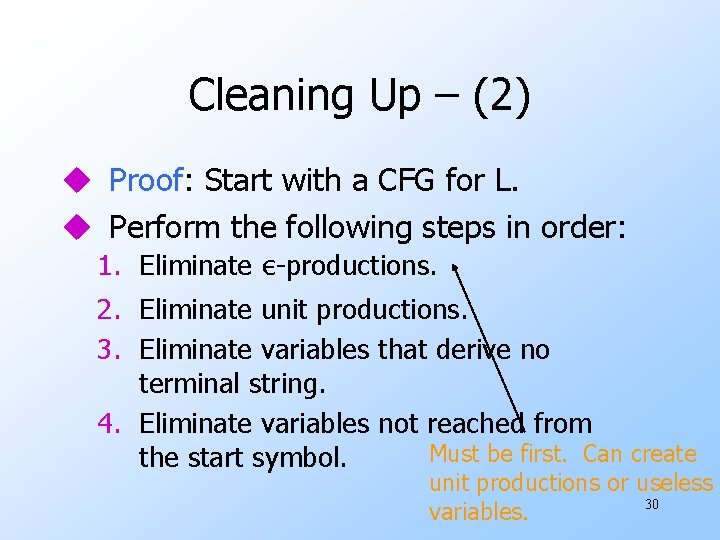Cleaning Up – (2) u Proof: Start with a CFG for L. u Perform the following steps in order: 1. Eliminate ε-productions. 2. Eliminate unit productions. 3. Eliminate variables that derive no terminal string. 4. Eliminate variables not reached from Must be first. Can create the start symbol. unit productions or useless 30 variables.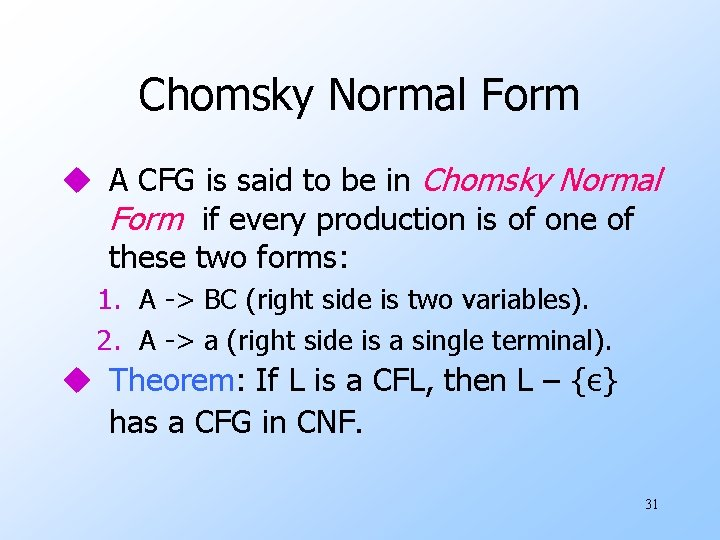Chomsky Normal Form u A CFG is said to be in Chomsky Normal Form if every production is of one of these two forms: 1. A -> BC (right side is two variables). 2. A -> a (right side is a single terminal). u Theorem: If L is a CFL, then L – {ε} has a CFG in CNF. 31Proof of CNF Theorem u. Step 1: “Clean” the grammar, so every production right side is either a single terminal or of length at least 2. u. Step 2: For each right side a single terminal, make the right side all variables. w For each terminal a create new variable Aa and production Aa -> a. w Replace a by Aa in right sides of length > 2. 32Example: Step 2 u. Consider production A -> Bc. De. u. We need variables Ac and Ae. with productions Ac -> c and Ae -> e. w Note: you create at most one variable for each terminal, and use it everywhere it is needed. u. Replace A -> Bc. De by A -> BAc. DAe. 33CNF Proof – Continued u. Step 3: Break right sides longer than 2 into a chain of productions with right sides of two variables. u. Example: A -> BCDE is replaced by A -> BF, F -> CG, and G -> DE. w F and G must be used nowhere else. 34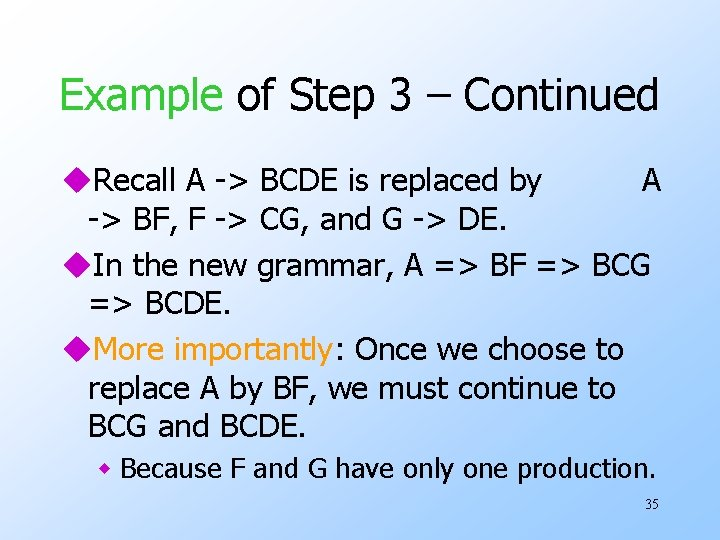Example of Step 3 – Continued u. Recall A -> BCDE is replaced by A -> BF, F -> CG, and G -> DE. u. In the new grammar, A => BF => BCG => BCDE. u. More importantly: Once we choose to replace A by BF, we must continue to BCG and BCDE. w Because F and G have only one production. 35CNF Proof – Concluded u. We must prove that Steps 2 and 3 produce new grammars whose languages are the same as the previous grammar. u. Proofs are of a familiar type and involve inductions on the lengths of derivations. 36